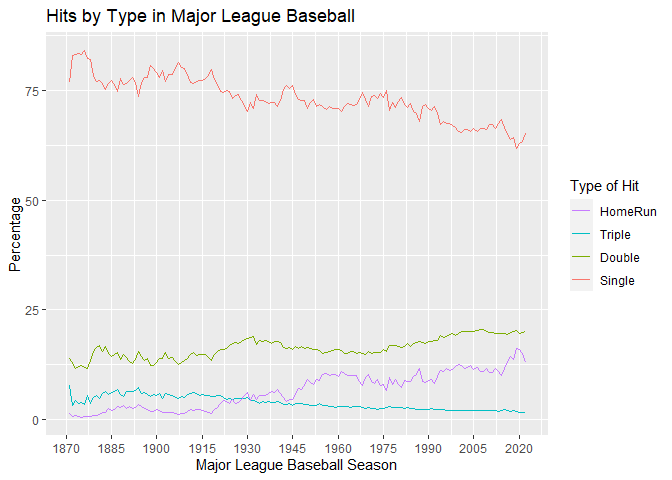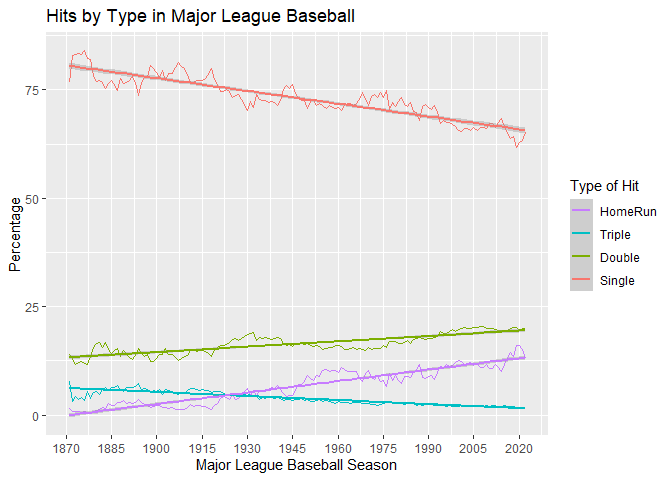# Graphs of Hits By Type in MLB

#### 2023-05-03

How has the percentage of various types of hits (singles, doubles, triples, home runs) changed over time in baseball history? Are there any overall trends? This vignette examines these questions in a simple analysis of the Batting data.

## The Batting data

First, we load the Batting data from the Lahman package. We also need to load the dplyr package so that we can sort and organize the data. The batting data has much more than we need.

library("dplyr")
data(Batting, package="Lahman")
str(Batting) #take a look at the data
## 'data.frame':    112184 obs. of  22 variables:
##  $playerID: chr "abercda01" "addybo01" "allisar01" "allisdo01" ... ##$ yearID  : int  1871 1871 1871 1871 1871 1871 1871 1871 1871 1871 ...
##  $stint : int 1 1 1 1 1 1 1 1 1 1 ... ##$ teamID  : Factor w/ 149 levels "ALT","ANA","ARI",..: 136 111 39 142 111 56 111 24 56 24 ...
##  $lgID : Factor w/ 7 levels "AA","AL","FL",..: 4 4 4 4 4 4 4 4 4 4 ... ##$ G       : int  1 25 29 27 25 12 1 31 1 18 ...
##  $AB : int 4 118 137 133 120 49 4 157 5 86 ... ##$ R       : int  0 30 28 28 29 9 0 66 1 13 ...
##  $H : int 0 32 40 44 39 11 1 63 1 13 ... ##$ X2B     : int  0 6 4 10 11 2 0 10 1 2 ...
##  $X3B : int 0 0 5 2 3 1 0 9 0 1 ... ##$ HR      : int  0 0 0 2 0 0 0 0 0 0 ...
##  $RBI : int 0 13 19 27 16 5 2 34 1 11 ... ##$ SB      : int  0 8 3 1 6 0 0 11 0 1 ...
##  $CS : int 0 1 1 1 2 1 0 6 0 0 ... ##$ BB      : int  0 4 2 0 2 0 1 13 0 0 ...
##  $SO : int 0 0 5 2 1 1 0 1 0 0 ... ##$ IBB     : int  NA NA NA NA NA NA NA NA NA NA ...
##  $HBP : int NA NA NA NA NA NA NA NA NA NA ... ##$ SH      : int  NA NA NA NA NA NA NA NA NA NA ...
##  $SF : int NA NA NA NA NA NA NA NA NA NA ... ##$ GIDP    : int  0 0 1 0 0 0 0 1 0 0 ...

### Data munging

We take the full Batting data frame and select what we need to use. We want a data frame that shows us the year, followed by total hits for that year, and then singles, doubles, triples and home runs.

Singles is not a column in this data frame, so we need to add it by taking total hits (H), and subtracting the other types of hits from it. The mutate function does the math for us and adds a column in.

batting <- Batting %>%
# select the variables that we want left after we filter the data
select(yearID, H, X2B, X3B, HR) %>%
# select the years from 1871+
filter(yearID >= 1871) %>%
group_by(yearID) %>%
#  summarise_each(funs(sum(., na.rm=TRUE))) %>%
summarise_all(funs(sum(., na.rm=TRUE))) %>%
# we summarize by year, and then na.rm takes care of 0's in the data
mutate(X1 = H-(X2B+X3B+HR)) %>% #create a column for singles
# we eventually want these as a percentage of hits, so we can do the math now
mutate(Single = X1/H*100) %>%
mutate(Double = X2B/H*100) %>%
mutate(Triple = X3B/H*100) %>%
mutate(HomeRun = HR/H*100)

Now, just select the variables we want to plot

bat <- batting %>%
select(yearID, Single, Double, Triple, HomeRun)
#this makes a nice looking data frame before we move on

We have our data in wide format right now. We need it to be in long format so that we can use ggplot to make a graph. The reshape2 package does this easily. We want to melt our data frame, but keep YearID as the ID variable (meaning that it stays put in it’s own column). Then, we look at the data to make sure it’s what we want.

library(reshape2)
bat_long <- melt(bat, id.vars = c("yearID"))
##   yearID variable value
## 1   1871   Single 76.78
## 2   1872   Single 82.92
## 3   1873   Single 83.19
## 4   1874   Single 83.38
## 5   1875   Single 83.09
## 6   1876   Single 84.00

## Graphs

To look at hits per year in a line graph, we will use ggplot2. The data is called bat_long, and our variables of interest are year (yearID), the percentage of each type of hit (value), and the type of hit (variable).

We can use geom_line and then make titles with xlab, ylab, and ggtitle. Instead of using the default scaling, we can set our own scale_x and scale_y.

The guides function tells ggplot what we want from our legend and overrides the default. We want singles at the bottom (so we reverse the legend which automatically does the opposite), and we want to set our own title for the legend.

library(ggplot2)
hitsperyear <- ggplot(bat_long, aes(x=yearID, y= value, col=variable)) +
geom_line() +
xlab("Major League Baseball Season") +
ylab("Percentage") +
ggtitle("Hits by Type in Major League Baseball") +
scale_x_continuous(breaks = c(1870, 1885, 1900, 1915, 1930, 1945,
1960, 1975, 1990, 2005, 2020 )) +
scale_y_continuous(breaks = c(0, 25, 50, 75, 100))+
guides(colour=guide_legend(reverse=TRUE,
aes(ggtitle= "Type of Hit")))
hitsperyearWe can see the overall trends more clearly by adding linear regression lines for each type of hit.

hitsperyear + geom_smooth(method="lm")So, the percentage of singles and triples have declined over time, while the percentage of doubles and home runs have increased. Can you think of any reason for this?

• Would it make sense to exclude players who have fewer than some number of plate appearances (AB) in a given year?